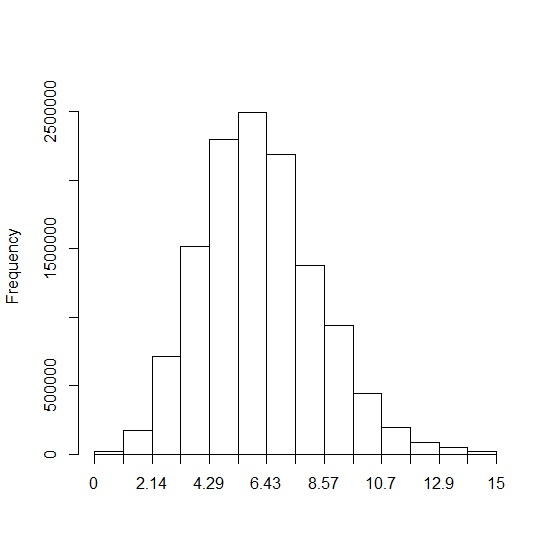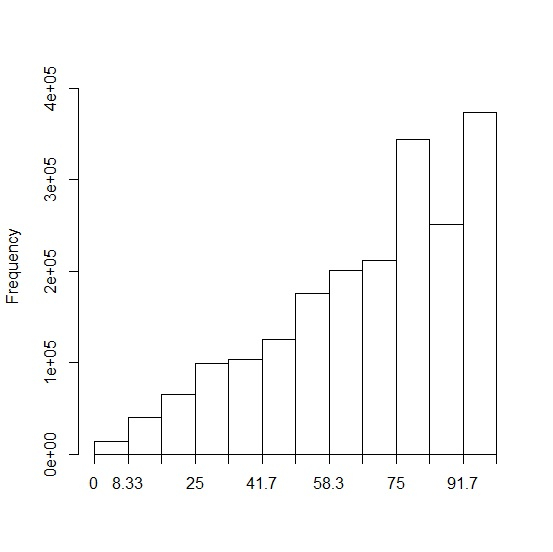# How to create a histogram using weights in R?

A histogram using weights represent the weighted distribution of the values. In R, we can use weighted.hist function of plotrix package to create this type of histogram and we just need the values and weights corresponding to each value. Since plotrix is not frequently used, we must make sure that we install this package using install.packages("plotrix") then load it in R environment.

library("plotrix")

Consider the below vector and the weight associated with that vector −

## Example

x<-sort(rpois(5000,5))
weight<-seq(1,5000)

Creating weighted histogram for x −

## OutputLet’s have a look at another example −

## Example

y<-sort(sample(0:100,2000,replace=TRUE))
weight<-seq(1,2000) \
weighted.hist(y,weight)

## Output